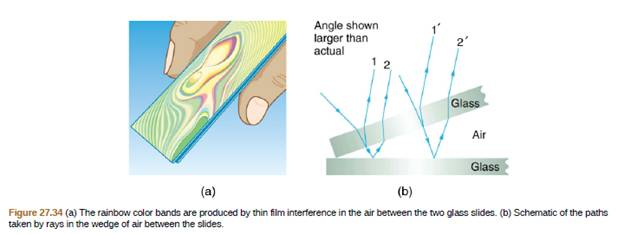Chapter 27, Problem 79PE

Chapter
Section
Textbook Problem

Figure 27.34 shows two glass slides illuminated by pure-wavelength light incident perpendicularly. The top slide touches the bottom slide at one end and rests on a 0.100-mm-diameter hair at the other end, forming a wedge of air. (a) How far apart are the dark bands, if the slides are 7.50 cm long and 589-nm light is used? (b) Is there any difference if the slides are made from crown or flint glass? Explain.To determine

(a)

How far apart are the dark bands?

Explanation

Given info:

Diameter of the hair, d=0.1mm

Length of the slide, l=7.50cm

Wavelength of the light, λ=589nm

Formula used:

The distance of the slide is calculated by the formula

dsinθ=λ

Here,

λ is the wavelength

d is the distance of the slide

θ is the angle between glass slide

Calculation:

The angle between the glass slides is calculated by the formula

tanθ=dia.ofthehairlengthoftheslide

Plugging the values in the above equation

θ=tan10

To determine

(b)

Is there any difference if the slides are made from crown or flint glass?

Still sussing out bartleby?

Check out a sample textbook solution.

See a sample solution

The Solution to Your Study Problems

Bartleby provides explanations to thousands of textbook problems written by our experts, many with advanced degrees!

Get Started

What are genomes?

Human Heredity: Principles and Issues (MindTap Course List)

4. The normal arterial range is

Cardiopulmonary Anatomy & Physiology

Match the term listed in Column A with its definition from Column B

Nutrition Through the Life Cycle (MindTap Course List)